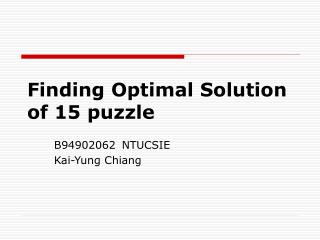# Finding Optimal Solution of 15 puzzle - PowerPoint PPT PresentationDownload PresentationFinding Optimal Solution of 15 puzzle

Finding Optimal Solution of 15 puzzle
Download Presentation## Finding Optimal Solution of 15 puzzle

- - - - - - - - - - - - - - - - - - - - - - - - - - - E N D - - - - - - - - - - - - - - - - - - - - - - - - - - -
##### Presentation Transcript

1. Finding Optimal Solution of 15 puzzle B94902062 NTUCSIE Kai-Yung Chiang

2. 15 puzzle introduction Initial states: a solvable puzzle, including numbered 1~15 tiles and a blank tile 0 Goal state: puzzle in which tiles 0 ~ 15 are in well permutation from row to column A (valid) move: swap blank tile with its neighboring tile The optimal solution: given a initial state, find the move sequence such that it is a solution taking the fewest moves to reach the goal state

3. Searching Algorithm A* search Each node has heuristic (the estimating distance from current state to goal state) Each node’s evaluation function = heuristic + total moves from initial state A* always expands the fringe node whose evaluation function is minimum

4. : fringe nodes : expanded nodes h: heuristic f: evaluation function Example h=45, f=45

5. : fringe nodes : expanded nodes h: heuristic f: evaluation function Example h=45, f=45 h=41, f=42 h=45,f=46 h=42, f=43

6. : fringe nodes : expanded nodes h: heuristic f: evaluation function Example h=45, f=45 h=41, f=42 h=45,f=46 h=42, f=43 h=45, f=47 h=42, f=44 h=43, f=45

7. : fringe nodes : expanded nodes h: heuristic f: evaluation function Example h=45, f=45 h=41, f=42 h=45,f=46 h=42, f=43 h=45, f=47 h=42, f=44 h=43, f=45 h=40,f=42 h=39, f=41 h=45,f=47

8. Pros and cons for A* search • Pros: • Can find the optimal solution (or admissible) if heuristic function will never overestimate • Eliminate number of expanded nodes • Cons: • Taking more time on expanding each nodes (compared with DFS or BFS): • Find minimum nodes (in fringe) to expand • Compute heuristic and evaluation function • Check, eliminate and update repetitive states • Maintain data structure

9. Speed up by good data structure Priority queue: Fibonacci heap Extract minimum and decrease key in (amortized) O(logn) Insert in O(1) Hash function: Used in checking repetitive states, eliminate number of searching nodes A* search with above data structure and Manhattan distance as heuristic function could solve 8 puzzle in (averaged) 0.002 seconds However, still too slow to solve 15 puzzle

10. More speed up technique • Still too many nodes need to be expanded (though eliminate repetitive states) • If f(n) = 2h(n) + moves(n)… • Could speed up much, but not admissible. • Ideas: try to improve h(n) to be more close to truly needed optimal moves, while still maintaining admissible • Disjoint pattern database heuristic instead of Manhattan distance • New heuristic = ∑i number of needed moves to well order tiles in pattern i

11. Pattern database • Divide puzzle into 3 disjoint patterns • Each pattern has 16!/10!≒5.7 * 106 different combination orders of tiles, and each of them corresponds to a database entry • Each entry records moves for aligning these pattern tiles to correct position

12. Building pattern database • Constructed by bottom up approach • Start with well ordered tiles • BFS moving blank tile to new states, move is added only when tile in this pattern is swapped with blank tile • Add new entry to pattern database if current state has not been traversed (using a bits of array to record this information)

13. Benchmark and outcome • Randomly generate 100 solvable puzzles:

14. Benchmark and outcome • Pattern database heuristic improves nearly 10 moves than Manhattan distance in averaged • In most problem instances… • Expanded nodes are less than 100,000 • Could be solved in 30 seconds, and more than half could be solved in 10 seconds • CPU% are less than 20%. It might suggest that lots of time are took in communication with database • Pattern database heuristic improves a lot